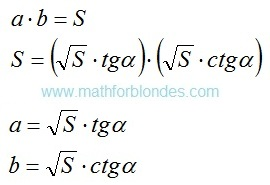## 9/03/2016

### The linear angular functions

Subject of occupations:
TRIGONOMETRIC FUNCTIONS IN A RECTANGLE
Subject of the previous lesson
Division

Lesson 14

The linear angular functions

If to consider terminating trigonometric functions as coordinates of points of a unit circle in a Cartesian coordinate system, then the linear angular functions are coordinates of points of the chord connecting circle cross points to a coordinate. The sum of coordinates of any point of this chord is always equal to unit.The linear angular functions

In mathematician of a concept, similar to the linear angular functions, are used since ancient times - it is division whole on a part. In the modern world an analog are percent.

The explanation for readers of this website. For myself I called the linear angular functions "linos" and "loses". How I thought up these names? Took designation of a sine and cosine. Visually they quite well differ. In each designation I replaced the first letter with the Latin letter "l" from the word "line". It turned out quite nicely. But to solve to you. Whether these functions in mathematician will get accustomed and as they will be called - time will show. I just offer one more mathematical tool for the description of reality.

At the following lesson we will consider

### Division

Subject of occupations:
TRIGONOMETRIC FUNCTIONS IN A RECTANGLE
Subject of the previous lesson
Decomposition on factors

Lesson 13

Division

Contrary to the standard opinion, division is not mathematical operation. This solution of a standard task of finding of one of factors if other factor and result of multiplication is known. In ancient Babylon the fraction was considered as result of multiplication of number to inverse other number. Even in the modern mathematics there is no division of one fraction into other fraction, this operation is replaced with multiplication of a dividend to fraction, inverse to a divider.

Division can be considered as a projection of result of multiplication along one of factors. For example, length is a projection of the area along width, width is a projection of the area along length.

The most interesting in this plan is the speed which is measured by the lengthiest, divided into time. If to assume that length is result of multiplication of two perpendicular directions of time, then speed is a projection of length (the area of time) along one of the directions of time. For a comprehension of the nature and an essence of light velocity, this approach can be the very useful.

At the following lesson we will consider
The linear angular functions

### Decomposition on factors

Subject of occupations:
TRIGONOMETRIC FUNCTIONS IN A RECTANGLE
Subject of the previous lesson
Zero and infinity

Lesson 12

Decomposition on factors

Mathematical operation, opposite on sense to multiplication, decomposition on factors is. It is carried out with application of the infinite trigonometric functions.Decomposition on factors
The most prime example of decomposition on factors at an angle in 45 degrees is root squaring. As both factors in this case are identical, as result of decomposition it is accepted to write down only one of factors.

Decomposition on factors can be applied when only the result of multiplication is known and any of factors is not known. Units of measure as a result decomposition on factors should be selected intuitively so that as a result of their multiplication the tentative unit of measure turned out. The quantity of spacelike dimensions in units of measure of factors at decomposition can be a miscellaneous. For example, three-dimensional volume can be spread out to one-dimensional factors by means of two operations of decomposition, one of options looks so:Decomposition of volume

In this example corners α and β are not bound among themselves. If to display volume in a cube (a=b=c), then α≈35° is a angle between the diagonal of a cube and diagonal of the basis, β=45 ° is a angle between the diagonal of the basis and its party.

At the following lesson we will consider
Division